Home > A2C > Chapter 2 > Lesson 2.1.1 > Problem2-12

2-12.
1. Carmel wants to become a “Fraction Master.” He has come to you for instruction. Homework Help ✎

1. Help Carmel by demonstrating and explaining every step necessary to simplify the problem below.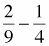2. Oh no!” exclaimed Carmel. “This one is hard!” Show him every step he needs to simplify the problem below. (Note that from this point on in the course, you may assume that all values of a variable that would make a denominator zero are excluded.)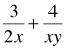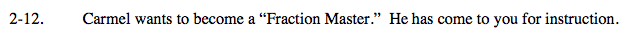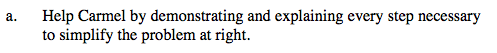Change to common denominator.

$\frac{8}{36}-\frac{9}{36}$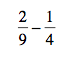Now just subtract the fractions.

$-\frac{1}{36}$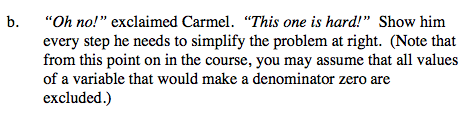Change to common denominator. (2xy)

$\frac{3\textit{y}}{2\textit{x}\textit{y}}+\frac{8}{2\textit{x}\textit{y}}$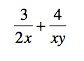Add the fractions to get one fraction.

$\frac{3\textit{y}+8}{2\textit{x}\textit{y}}$